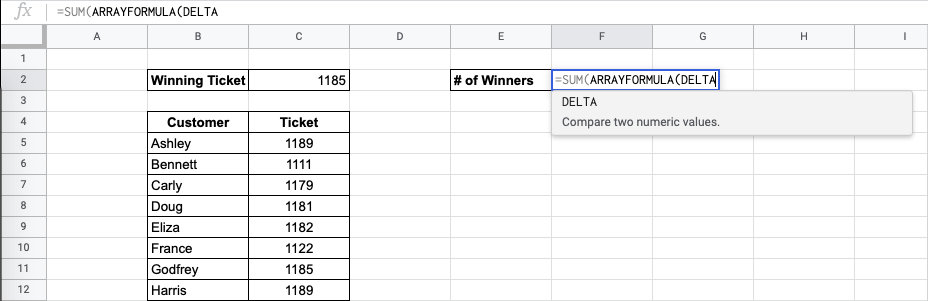# How to Use DELTA Function in Google Sheets

The DELTA function in Google Sheets is used to compare two numeric values, and returns the value ‘1’ if they are equal.

This function is useful because it helps you to immediately see if two given numbers are equal or not. This is an engineering function, inspired and named after the Kronecker delta function of two variables which are non-negative integers.

The function keeps everything in your spreadsheet straightforward and understandable for new users. This will avoid broken formulas and mismatched information in your spreadsheets at the end of the day, which will make you run into some difficulties with your overall data in the long run.

Let’s look at an example.

You have a spreadsheet that details the scores that some people have reported on their modules, but you also have a record of the same module. You want to go through the whole class and see if the reported and actual scores are the same.

The `DELTA` function takes in the two inputs and determines if they are equal or not. Instead of scanning the entire spreadsheet and using arbitrary formulas that offer no explanation, this is a helpful tool that will allow for such a calculation to take place in a neat manner.

## The Anatomy of DELTA Function in Google Sheets

The syntax of the `DELTA` function is as follows:

`=DELTA(number1, [number2])`

Let’s have a look at each part of the function to understand what is going on here:

• `=` is the equals sign that starts off any function in Google Sheets.
• `DELTA` is the name of our function.
• `number1` is the first value to be compared.
• `[number2]` is the optional second value to be compared. If it’s not provided, its default is zero.

Note that if you do not provide a `[number2]`, then the `number1` will be compared to 0 (zero).

This function can only compare numbers. So if there are other values you need to compare, you can use the `EQ` function.

## A Real Example of Using DELTA Function

You may be curious as to why the `DELTA` function exists, when there are other ways to compare two values.

The `DELTA` function is an engineering function. It helps to create conditions in Google Sheets to make it easy to follow algebraic equations. It is helpful to have a function that returns a certain value (in this case, 1) should two values be equal. This is helpful in a variety of very specific tasks.

Let’s look at the example below to see how to use the `DELTA` function in Google Sheets.

#### Viewing the matching numbers in Google Sheets

This is a simple problem. It is a simplified task to help you understand what the function can be used for.The function takesup to 2 arguments. So in the equation, it will look like:

```=DELTA(C5,\$C\$2)
```

Note that we added dollar signs to lock the value in the Winning Ticket cell. Of course, we want to return “WIN” or “LOSE” so we proceed with the following formula:

`=IF(DELTA(C5,\$C\$2)=1,"WIN","LOSE")`

You should now see a helpful list that will allow you to sort through the winners and losers of the draw quickly. This simple problem can be practiced to perfection. Use the link below to get a copy of this problem set:

## How to Use DELTA Function in Google Sheets

In this section, we will show you a step-by-step process on how to use the `DELTA` function in Google Sheets.

In this problem, we will find the number of winners in the entire draw.

#### Using DELTA Function within Formulas In Google Sheets

1. To begin, prepare your spreadsheet with the information you want to check.1. Start to fill in the `SUM` function. This is because we want to find the total number of winners.1. Start to fill in the `ARRAYFORMULA` function. This function helps enable the display of values returned from an array formula into multiple rows or columns and the use of non-array functions with arrays. Some functions in Google Sheets are not flexible enough to work with a range.`ARRAYFORMULA` function helps with this.1. Start to fill in the `DELTA` function.1. Choose the proper range. We can do this because we are using Arrayformula. Chose the starting cell, C5, then stretch the range to the rest of the C column. Note that any other values that are written in C will count towards the formula. So if you add new entries, they will be accounted for in the formula.1. Next, click on the value we are comparing the individual ticket numbers to.1. This formula is now done, so close the formula. You can notice you have a preview. You’re now done!The `DELTA` function can help tidy up your spreadsheet. This should definitely help you avoid mistakes in your spreadsheets in the future, too.

And there you have it – you can now use the `DELTA` function in Google Sheets together with the other numerous Google Sheets formulas to create even more effective formulas.Our goal this year is to create lots of rich, bite-sized tutorials for Google Sheets users like you. If you liked this one, you'll love what we are working on! Readers receive ✨ early access ✨ to new content. There will be no spam and you can unsubscribe at any time.

##### You May Also Like## How to Use XNPV Function in Google Sheets

The XNPV function in Google Sheets is a useful tool to help determine the net present value of…## How to Use TODAY Function in Google Sheets

The TODAY function in Google Sheets is useful to return the current date. We can use the TODAY…## How to Use WEEKDAY Function in Google Sheets

The WEEKDAY function in Google Sheets is useful if you want to give a numerical value to a…## How to Use LOWER Function in Google Sheets

The LOWER function in Google Sheets is useful if you want to convert a given text into small…